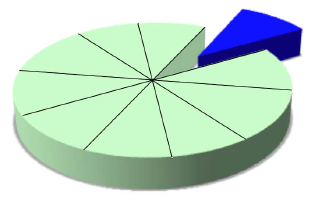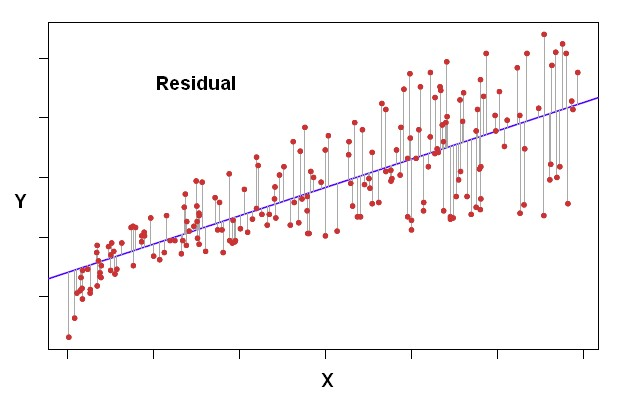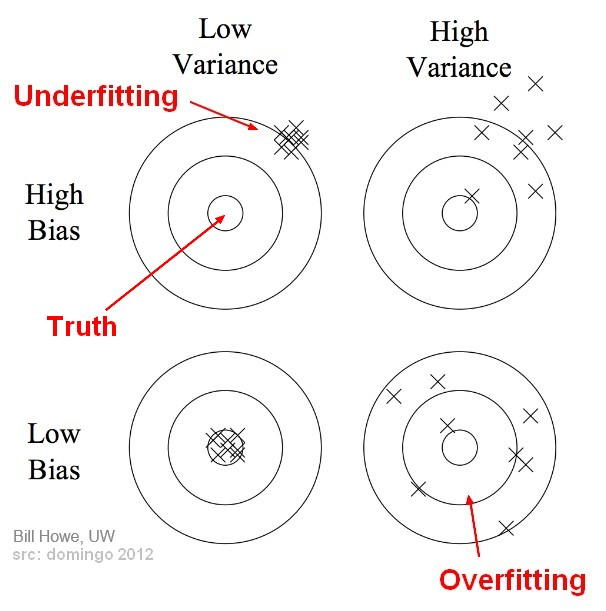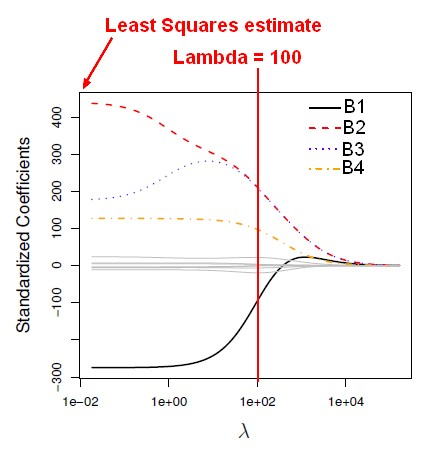# Statistics - Bias-variance trade-off (between overfitting and underfitting)

The bias-variance trade-off is the point where we are adding just noise by adding model complexity (flexibility). The training error goes down as it has to, but the test error is starting to go up. The model after the bias trade-off begins to overfit.

When the nature_of_the_problem is changing the trade-off is changing.

## Formula

The ingredients of prediction error are actually:

• bias: the bias is how far off on the average the model is from the truth.
• and variance. The variance is how much that the estimate varies around its average.

Bias and variance together gives us prediction error.

This difference can be expressed in term of variance and bias:

$e^2 = var(model) + var(chance) + bias$

where:

• $var(model)$ is the variance due to the training data set selected. (Reducible)
• $var(chance)$ is the variance due to chance (Not reducible)
• bias is the average of all $\hat{Y}$ over all training data set minus the true Y (Reducible)

As the flexibility (order in complexity) of f increases, its variance increases, and its bias decreases. So choosing the flexibility based on average test error amounts to a bias-variance trade-o ff.

## Illustration

where:

We want to find the model complexity that gives the smallest test error.

When the nature_of_the_problem is changing the trade-off is changing.

## Nature of the problem

When the nature of the problem is changing the trade-off is changing.

• the truth is wiggly and the noise is high, so the quadratic do the best
• the truth is smoother, so the linear model do really well
• the truth is wiggly and the noise is low, so the more flexible do the best

## Model Complexity is better/worse

Model Complexity = Flexibility

• The sample size is extremely large, and the number of predictors is small: Flexible is better. A flexible model will allow us to take full advantage of our large sample size.
• The number of predictors is extremely large, and the sample size is small: Flexible is worse. The flexible model will cause overfitting due to our small sample size.
• The relationship between the predictors and response is highly non-linear. A flexible model will be necessary to find the nonlinear effect.
• The variance of the error terms, i.e. sigma^2 = var(Epsilon) , is extremely high: Flexible is worse. A flexible model will cause us to fit too much of the noise in the problem.

## Documentation / Reference

Recommended Pages(Statistics|Data Mining) - (K-Fold) Cross-validation (rotation estimation)

Cross-validation, sometimes called rotation estimation is a resampling validation technique for assessing how the results of a statistical analysis will generalize to an independent new data set. This...Data Mining - (Function|Model)

The model is the function, equation, algorithm that predicts an outcome value from one of several predictors. During the training process, the models are build. A model uses a logic and one of several...Machine Learning - (Overfitting|Overtraining|Robust|Generalization) (Underfitting)

A learning algorithm is said to overfit if it is: more accurate in fitting known data (ie training data) (hindsight) but less accurate in predicting new data (ie test data) (foresight) Ie the model...Statistics - (Residual|Error Term|Prediction error|Deviation) (e| )

The residual is a deviation score measure of prediction error in case of regression. The difference between an observed target and a predicted target in a regression analysis is known as the residual...Statistics - (Variance|Dispersion|Mean Square) (MS)

The variance shows how widespread the individuals are from the average. The variance is how much that the estimate varies around its average. It's a measure of consistency. A very large variance means...Statistics - Bias (Sampling error)

Bias in stats Bias is a systematic error in sampling or measurement. Systematic measurement error represents bias. It has an effect on the entire distribution (It shift it right or left). The bias...Statistics - Ridge regression

Ridge regression is a shrinkage method. It was invented in the '70s. The least squares fitting procedure estimates the regression parameters using the values that minimize RSS. In contrast, the...